Function Repository Resource:

# GergonnePoint

Return the Gergonne point of a triangle

Contributed by: Shenghui Yang
 ResourceFunction["GergonnePoint"][{p1,p2,p3}] returns the Gergonne point of the triangle defined by vertices p1,p2 and p3.

## Details

ResourceFunction["GergonnePoint"] is the perspector of a triangle and its contact triangle.
The existence of the Gergonne point follows directly from Ceva's theorem.
ResourceFunction["GergonnePoint"] effectively uses the corresponding triangle center function to compute barycentric coordinates.
ResourceFunction["GergonnePoint"][Triangle[{p1,p2,p3}]] is equivalent to ResourceFunction["GergonnePoint"][{p1,p2,p3}].

## Examples

### Basic Examples (2)

Find the Gergonne point of three triangle vertices:

 In:=Out=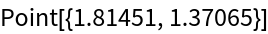The perspectors concur at the Gergonne point (marked Ge in the diagram):

 In:=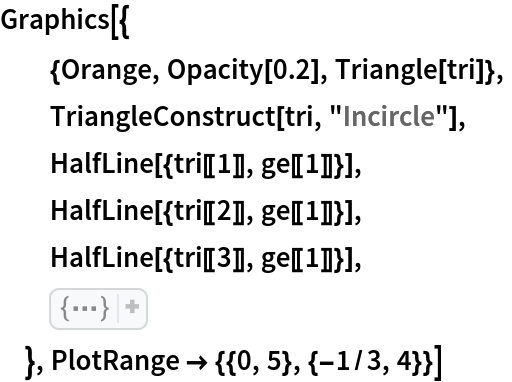Out=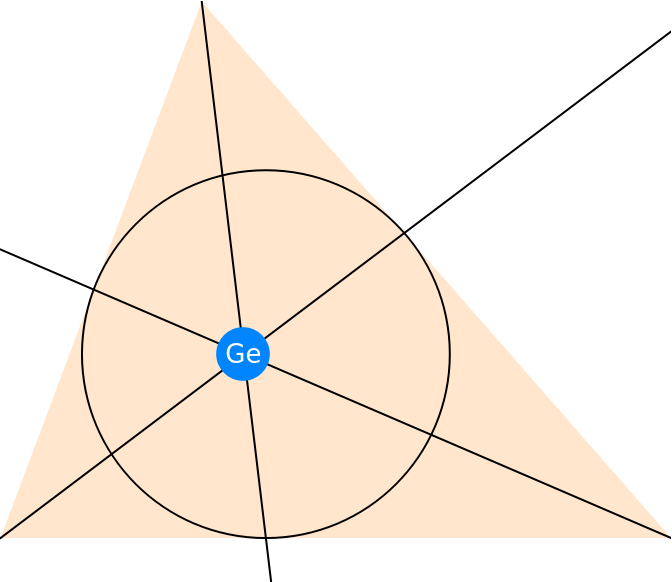### Scope (2)

Compute the Gergonne point of a 3D triangle:

 In:=Out=Show the Gergonne point and the triangle together:

 In:=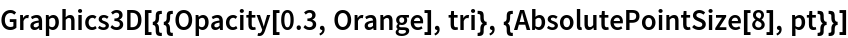Out=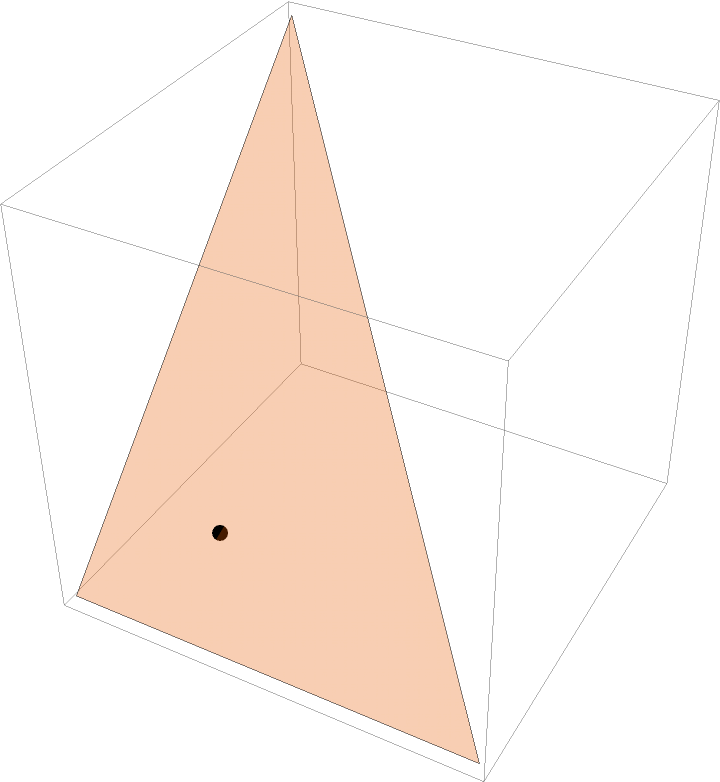### Neat Examples (3)

The Gergonne point, incenter and de Longchamps point (Kimberling center X20) are collinear:

 In:=In:=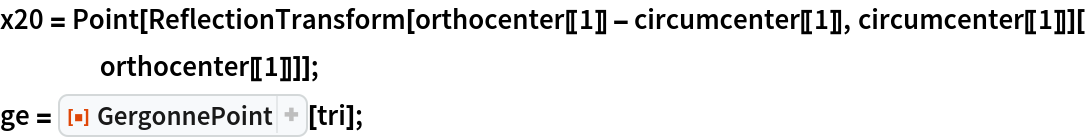Show the collinearity:

 In:=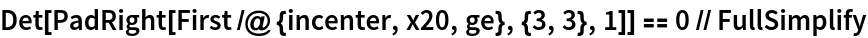Out=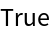Visualize the points and the line passing them:

 In:=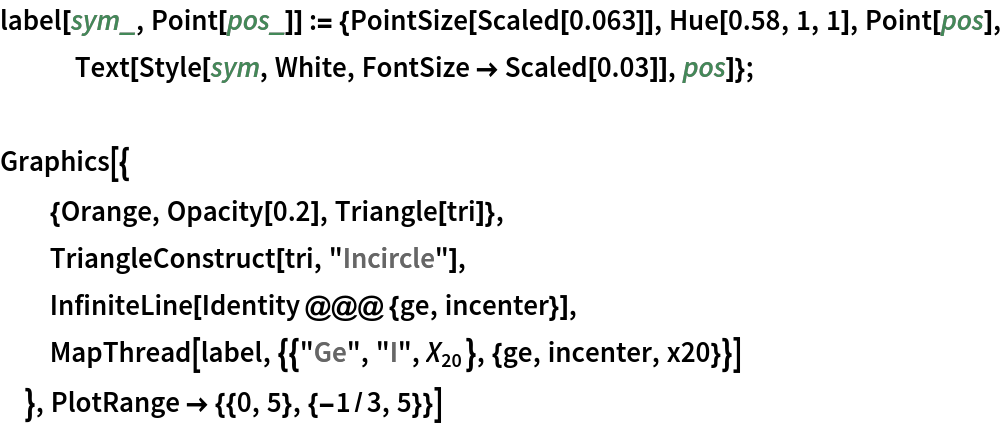Out=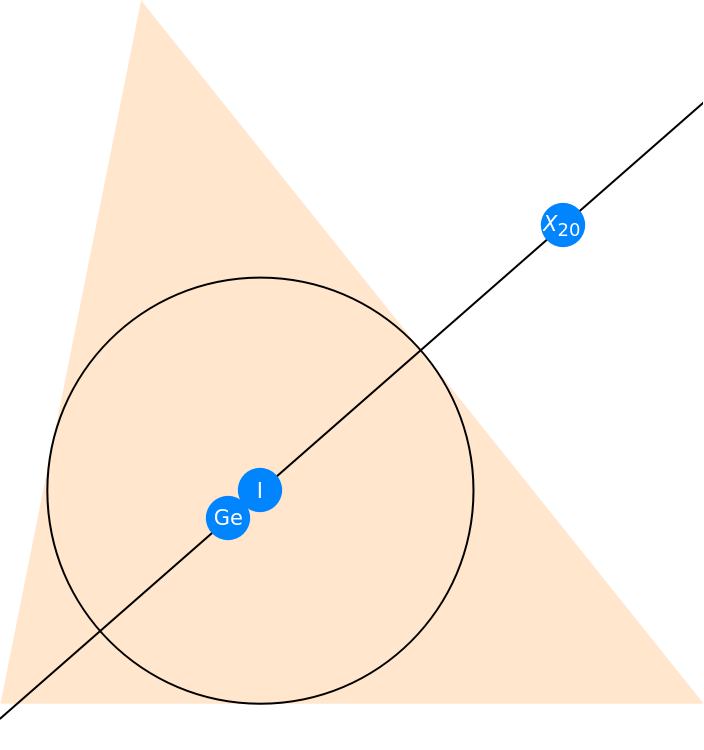Shenghui Yang

## Version History

• 1.0.0 – 16 August 2021